﻿ 烟草DNA序列分组测量可视化分析

# 烟草DNA序列分组测量可视化分析 The Visual Analysis of Packet Measurement about Tobacco DNA Sequence

Abstract: With the development of genetic engineering technology, the gene database is expanding, and the methods of DNA data processing are improving. In this paper, the complex tobacco DNA data are projected into a visualized graph by using the variable map technique. And the data are projected separately using different segments. These results provide a reference for further tobacco genome research.

1. 引言

2. 系统架构

2.1. 架构

2.2. 处理模块

2.3. 投影模块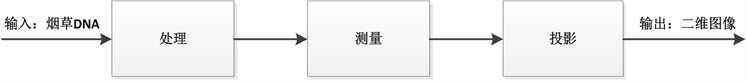Figure 1. Process module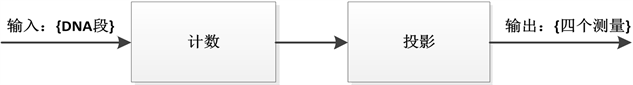Figure 2. Projection module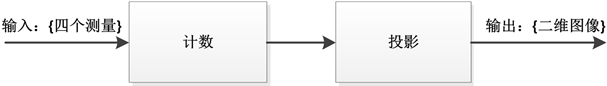Figure 3. Measuring module

2.4. 测量模块

3. 可视化结果及分析

3.1. 参数解释

m：每组处理DNA序列的长度(这里我们分别选取了m = 80，100，120，140，160，180，200，220，240，260段长度做可视化处理)；

V为AT，AG两个碱基组中的一个，V∈{AT, AG}；

PV为碱基组的比例；

Result1、Result2：分别保存DNA序列中AT数量、AG数量的数组；

Result1 = NUM(A) + NUM(T)；

Result2 = NUM(A) + NUM(G)；

Result1(x)：表示第x组中A的个数与T的个数之和；

Result2(y)：表示第y组中A的个数与G的个数之和；

(PAT, PAG) = (Result1(x), Result2(y))映射生成图像上的点。

3.2. 不同分段的投影图像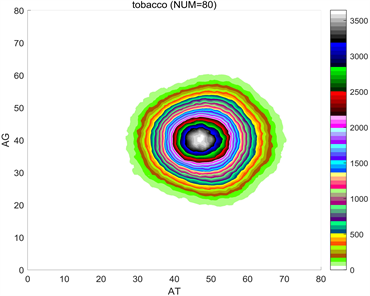(a) m = 80(b) m = 100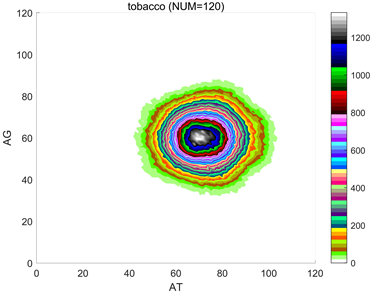(c) m = 120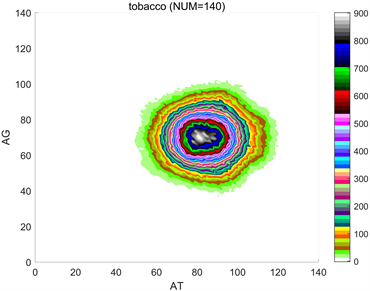(d) m = 140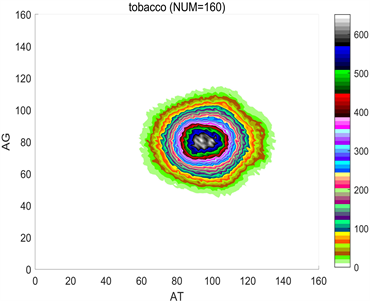(e) m = 160(f) m = 180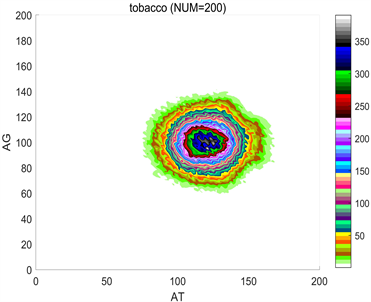(g) m = 200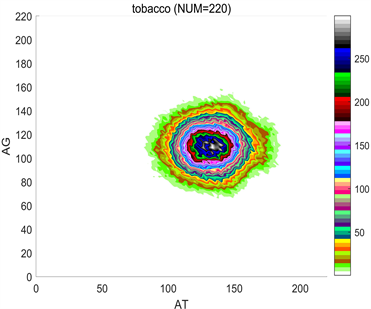(h) m = 220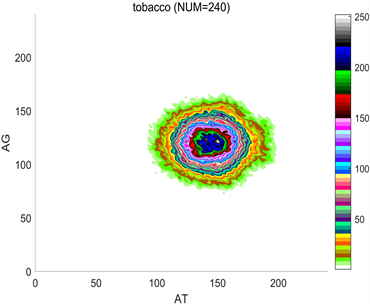(i) m = 240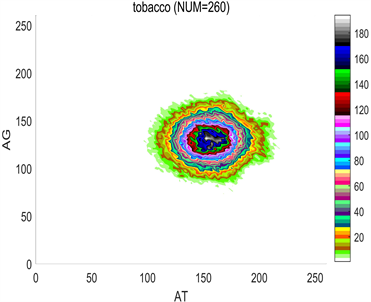(j) m = 260

Figure 4. Color maps of different group lengths (m = 80~260)

3.3. 简要分析

4. 总结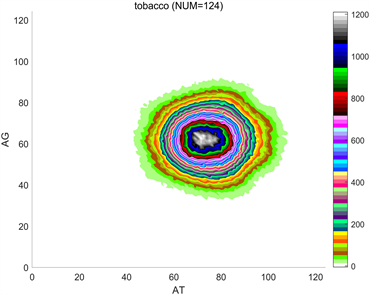(a) m = 124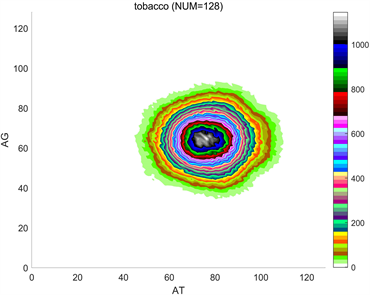(b) m = 128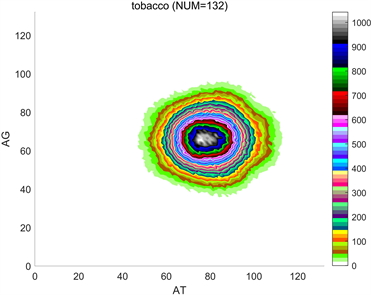(c) m = 132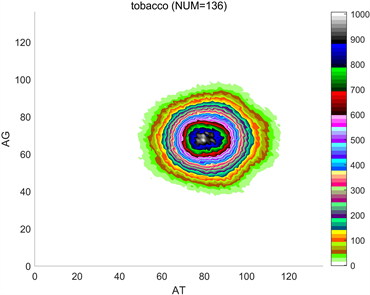(d) m = 136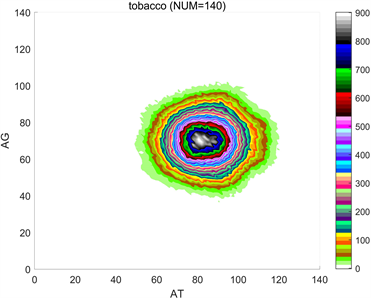(e) m = 140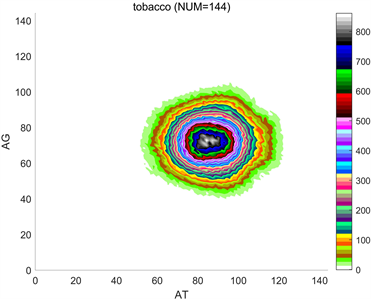(f) m = 144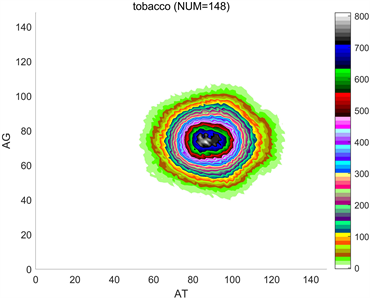(g) m = 148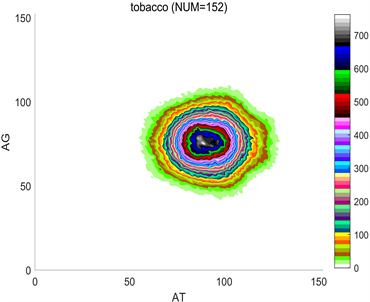(h) m = 152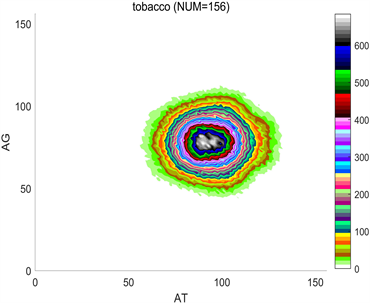(i) m = 148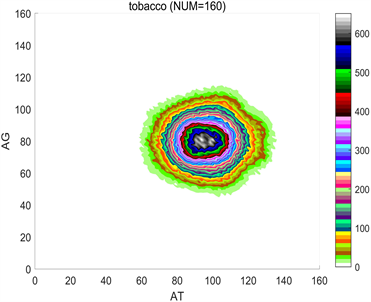(j) m = 152

Figure 5. Color maps of different group lengths (m = 124~160)

 Berger, J.A., Mitra, S.K., Carli, M. and Neri, A. (2004) Visualization and Analysis of DNA Sequences Using DNA Walks. Journal of the Franklin Institute, 341, 37-53.
https://doi.org/10.1016/j.jfranklin.2003.12.002

 Pitt, J.N., Rajapakse, I. and Ferre-D’Amare, A.R. (2010) SEWAL: An Open-Source Platform for Next-Generation Sequence Analysis and Visualization. PMC, 38, 7908-7915.
https://doi.org/10.1093/nar/gkq661

 Bernstein, B.E., Birney, E., Dunham, I., et al. (2012) An Integrated Encyclopedia of DNA Elements in the Human Genome. Nature, 489, 57-74.
https://doi.org/10.1038/nature11247

 Pennisi, E. (2012) Genomics. ENCODE Project Writes Eulogy for Junk DNA. Science, 337, 1159-1161.
https://doi.org/10.1126/science.337.6099.1159

 Li, Q.P. and Zheng, Z.J. (2010) Spatial Distributions for Measures of Random Sequences Using 2D Conjugate Maps. Proceedings of Asia-Pacific Youth Conference on Com-munication, Kunming, 64-69.

 张巍琼, 郑智捷. 基于不同产生机制的伪随机序列和DNA序列的随机性测量[J]. 成都信息工程学院学报, 2012, 27(6): 548-555.

 刘玉倩, 郑智捷. 编码和非编码DNA序列的可视化分析[J]. 计算生物学, 2014, 4(2): 20-31.

 完竹, 郑智捷. DNA序列一维分段测量分布可视化[J]. 云南大学学报(自然科学版), 2013(35): 1-6.

 杜磊, 郑智捷. 在非线性函数下的DNA概率测量聚类分布[J]. 软件工程与应用, 2014, 3(3): 41-49.

 李彦平, 丁燕芳, 孙焕, 李雪君, 朱景伟, 段旺军, 马浩波, 郭芳阳. 我国烟草育种现状及思考[J]. 河南农业科学, 2010(9): 148-150+156.

 Xu, S., Brockmöller, T., Na-varro-Quezada, A., et al. (2017) Wild Tobacco Genomes Reveal the Evolution of Nicotine Biosynthesis. Proceedings of the National Academy of Sciences, 114, 6133-6138.
https://doi.org/10.1073/pnas.1700073114

Top